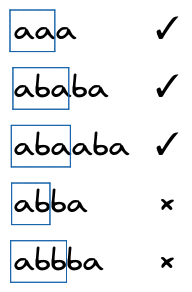# Wavio PalindromeMohaimin66The Tough Winter Spar, 20...
Limits 1s, 512 MB

Palindrome is a string which reads the same from both right to left and left to right. Formally, a string $s_1s_2...s_n$ of length $n$ is a palindrome if $s_i = s_{n-i+1}$, for all $i \leq \frac{n}{2}$.

Example: $a$, $aa$, $aba$, $cdadc$ are palindromes. But $ab$, $abb$, $cdbc$ are not palindromes.

Now let's define Wavio Palindrome. Palindromic string of length $n$ is a Wavio Palindrome if its prefix of length ⌈$\frac{n}{2}$⌉ is also a palindrome.

Example: $aaa$, $ababa$, $abaaba$ are Wavio Palindromes. But $abba$, $abbba$ are not.Given a string of length $l$, you have to count the number of palindromic substring of the given string having length greater than 2 and which is also a Wavio Palindrome. If any Wavio Palindromic substring occurs more than once in the given string, all its occurrences should be counted.

## Input

The first line is the number of test cases $T$. The first line of each test case contains an integer $l$, length of the input string. The next line of the test case is the input string $S$ of length $l$. It will consist of only lowercase English alphabet.

• $1\leq T \leq10$
• $1\leq l \leq1000000$

## Output

For each test case, print a line in the form "Case x: y" where $x$ is the case number and $y$ is the number of Wavio Palindromic substring in the input string.

## Sample

InputOutput
3
4
aaaa
8
abaabaaa
4
abab

Case 1: 3
Case 2: 2
Case 3: 0uDebug

### Submit